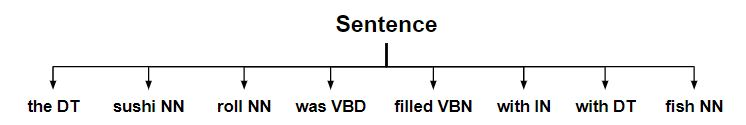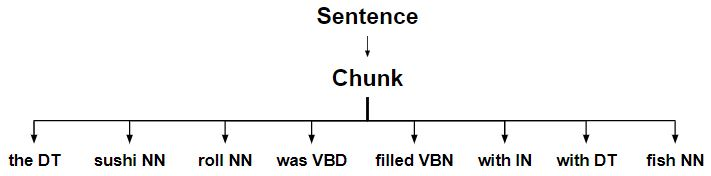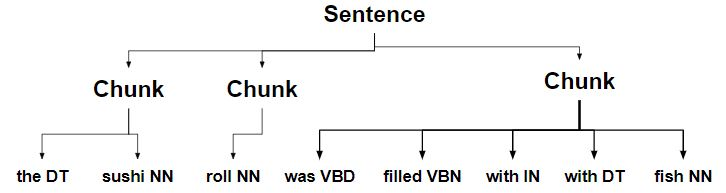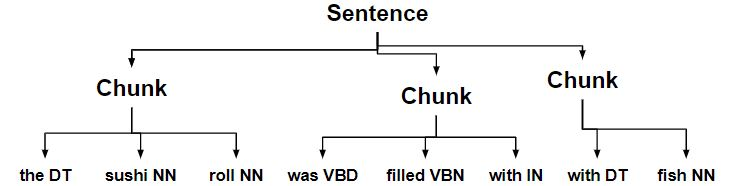# NLP | Splitting and Merging Chunks

SplitRule class : It splits a chunk based on the specified split pattern for the purpose. It is specified like <NN.*>}{<.*> i.e. two opposing curly braces surrounded by a pattern on either side.

MergeRule class : It merges two chunks together based on the ending of the first chunk and the beginning of the second chunk. It is specified like <NN.*>{}<.*> i.e. curly braces facing each other.

### Example of how the steps are performed

• Starting with the sentence tree.•

• Chunking complete sentence.•

• Chunks are split into multiple chunks.•

• Chunk with a determiner is split into separate chunks.•

• Chunks ending with a noun are merged with the next chunk.Code #1 – Constructing Tree

 `from` `nltk.chunk ``import` `RegexpParser ` `chunker ``=` `RegexpParser(r``''' ` `NP: ` `{
<.*>*} ` `}{<.*> ` `<.*>}{
` `{} ` `'''``) ` `sent ``=` `[(``'the'``, ``'DT'``), (``'sushi'``, ``'NN'``), (``'roll'``, ``'NN'``), (``'was'``, ``'VBD'``),  ` `        ``(``'filled'``, ``'VBN'``), (``'with'``, ``'IN'``), (``'the'``, ``'DT'``), (``'fish'``, ``'NN'``)] ` `chunker.parse(sent) `

Output:

```Tree('S', [Tree('NP', [('the', 'DT'), ('sushi', 'NN'), ('roll', 'NN')]),
Tree('NP', [('was', 'VBD'), ('filled', 'VBN'), ('with', 'IN')]),
Tree('NP', [('the', 'DT'), ('fish', 'NN')])])

```

Code #2 – Splitting and Merging

 `# Loading Libraries ` `from` `nltk.chunk.regexp ``import` `ChunkString, ChunkRule, ChinkRule ` `from` `nltk.tree ``import` `Tree ` `from` `nltk.chunk.regexp ``import` `MergeRule, SplitRule ` ` `  `# Chunk String ` `chunk_string ``=` `ChunkString(Tree(``'S'``, sent)) ` `print` `(``"Chunk String : "``, chunk_string) ` ` `  `# Applying Chunk Rule ` `ur ``=` `ChunkRule(``'
<.*>*'``, ``'chunk determiner to noun'``) ` `ur.``apply``(chunk_string) ` `print` `(``"\nApplied ChunkRule : "``, chunk_string) ` ` `  `# Splitting ` `sr1 ``=` `SplitRule(``''``, ``'<.*>'``, ``'split after noun'``) ` `sr1.``apply``(chunk_string) ` `print` `(``"\nSplitting Chunk String : "``, chunk_string) ` ` `  ` `  `sr2 ``=` `SplitRule(``'<.*>'``, ``'
'``, ``'split before determiner'``) ` `sr2.``apply``(chunk_string) ` `print` `(``"\nFurther Splitting Chunk String : "``, chunk_string) ` ` `  `# Merging ` `mr ``=` `MergeRule(``''``, ``''``, ``'merge nouns'``) ` `mr.``apply``(chunk_string) ` `print` `(``"\nMerging Chunk String : "``, chunk_string) ` ` `  `# Back to Tree ` `chunk_string.to_chunkstruct() `

Output:

```Chunk String :   <DT>  <NN>  <NN>  <VBD>  <VBN>  <IN>  <DT>  <NN>

Applied ChunkRule :  {<DT>  <NN>  <NN>  <VBD>  <VBN>  <IN>  <DT>  <NN>}

Splitting Chunk String :  {<DT>  <NN>}{<NN>}{<VBD>  <VBN>  <IN>  <DT>  <NN>}

Further Splitting Chunk String :  {<DT>  <NN>}{<NN>}{<VBD>  <VBN>  <IN>}{<DT>  <NN>}

Merging Chunk String :  {<DT>  <NN>  <NN>}{<VBD>  <VBN>  <IN>}{<DT>  <NN>}

Tree('S', [Tree('CHUNK', [('the', 'DT'), ('sushi', 'NN'), ('roll', 'NN')]),
Tree('CHUNK', [('was', 'VBD'), ('filled', 'VBN'), ('with', 'IN')]),
Tree('CHUNK', [('the', 'DT'), ('fish', 'NN')])])
```

Attention geek! Strengthen your foundations with the Python Programming Foundation Course and learn the basics.

To begin with, your interview preparations Enhance your Data Structures concepts with the Python DS Course.

My Personal Notes arrow_drop_upAspire to Inspire before I expire

If you like GeeksforGeeks and would like to contribute, you can also write an article using contribute.geeksforgeeks.org or mail your article to contribute@geeksforgeeks.org. See your article appearing on the GeeksforGeeks main page and help other Geeks.

Please Improve this article if you find anything incorrect by clicking on the "Improve Article" button below.

Article Tags :
Practice Tags :

Be the First to upvote.

Please write to us at contribute@geeksforgeeks.org to report any issue with the above content.